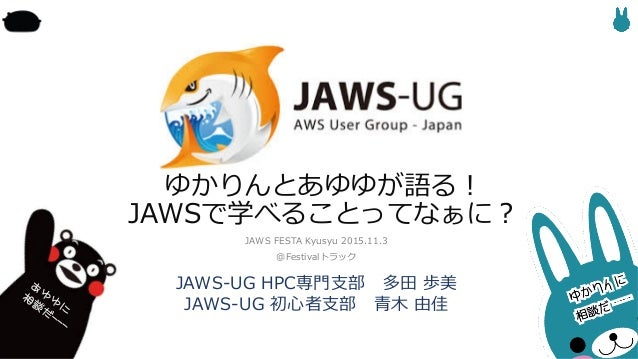Successfully reported this slideshow.Upcoming SlideShare
×

# JAWSFESTAKyusyu_JAWSで学べることってなぁに_ゆかりんあゆゆ_20151103

JAWS FESTA Kyusyu 20151103で、Festivalトラックで多田さんと青木がお話しました。キャリアのお話が入ってます！

• Full Name
Comment goes here.

Are you sure you want to Yes No### JAWSFESTAKyusyu_JAWSで学べることってなぁに_ゆかりんあゆゆ_20151103

1. 1. p B9LI o B9LI K? @G j B9LI K? j B9LI >=IJ9 Cfcafc ) . , 8>Tab dP
2. 2. u v B9LI K? r k fcZPOYf bT fcZP P Z 0.).
3. 3. j u v j B9LI K? @G ( ( j m m j u <9v 9LI I9EKH9A ) -‐‑‒ P_̲_̲ T TP OPfc Pfc bPSP /
4. 4. B9LI K? o BP_̲P u v 9LI KaT ? c_̲ 5B9LI K?u n r r rv B9LI K? wB9LI >=IJ9 l YPeac
5. 5. JAWS%UG YPeac .) I C T Wbb_̲3((YPea c Y_̲( o H 9LI 9LI l P DA
6. 6. pp w>Tab dP n k k r u v
7. 7. B9LI K? s s k AJ l uB9LI v u v DJ B9LI
8. 8. B9LI K? s s l ) 9LI l B9LI l T3A dT b ) , l B9LI <9MI ) -‐‑‒ p 9LI Ic b T3A dT bkB9LI <9MI k@G k
9. 9. T3A dT b k 9LI I9EKH9Ak@G k w 9LI 9LI k w
10. 10. w m t u <9 pv m t AJ r
11. 11. o m B9LI K? o
12. 12. o m9LI o o o
13. 13. o m o 9LI m (((
14. 14. o muB9LI K? FCv o
15. 15. u v k w k l y z l
16. 16. t l r u v t u j v
17. 17. w r pp r l t k l
18. 18. k l r w
19. 19. l B9LI >=IJ9 r
20. 20. B9LI >=IJ9 r p r JJ
21. 21. B9LI >=IJ9 r r u ov k k r
22. 22. w l y z r k l
23. 23. 9LI I9 ? T k l u v r r lll
24. 24. JAWS 9LI n9LI Ic b B9LI r r l
25. 25. AWS(Summit(TOKYO(2013 JAWS p w rrrppp w rrrrr p l
26. 26. JJ
27. 27. k o w k o m r e m J t mG m mw t m e
28. 28. k o w k o m? kJ u r w w v m r k l m lll u (((v
29. 29. (
30. 30. o w k o mw o j mB9LI r DJ uB9LI v j DJ
31. 31. o w o m T3A dT b ) , l m B9LI l m p m 9LI IP c P p m u lv
32. 32. k o w k k l
33. 33. ( ( (
34. 34. B9LI K? B9LI K? k 9LI o wB9LI K? w k YPeac O h"ps://jawsug,beginner.doorkeeper.jp/ h"p://jawsug,bgnr.blogspot.jp/
35. 35. B9LI K? @G 8 YPeac W_̲R ) . 1 l w @G p l k k r h"p://jawsug,hpc.connpass.com/8 h"ps://www.facebook.com/jawsughpc/h"p://www.slideshare.net/ayumitada126/20140628jawsughpc HPC
36. 36. B9LI K? R cS ) ppp B9LI K? l B9 u9gc T r r v k k k l r p h"p://www.slideshare.net/popowa/201507,jawsug,51302599 h"ps://cloudgirl.doorkeeper.jp/ h"ps://www.facebook.com/jawsug.cloudgirl h"p://cloudgirls.blogspot.jp/
37. 37. t w k
38. 38. k kw 64 pp w k k r r k r TbRt pp pp
39. 39. 9LI @K: o s 9LI @K:l s k r k 9LI @K:l >PRT Z k #ง i ว 9LI @K: >PRT Z r Wbb_̲a3((eee UPRT Z R (9ea@c 7U TU5ba
40. 40. y zB9LI K? B9LI <9MI ) .u,( v B9LI T3E bTu2(. v B9LI >=IJ9u p (, v 9LI cSH PSaW eu )( / k (-‐‑‒ k ( k ( v B9LI <9MI < ZTT_̲T k p B9LI K? r l Wbb_̲a3((YPea SPfa S ZTT_̲T Y_̲(
41. 41. m r m k r k u pv m k kw m tB KIppp
42. 42. B9LI K?